# Formula problem - adding values in the same line itemHi Guys,

I just want to ask help on the formula. I was able to create a formula and got the values but the succeeding time does not show no values.

This is what it looks like in excel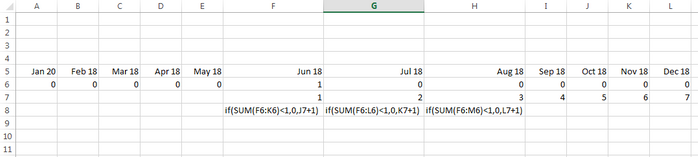I also included the formula on the sheet for col K, L and M. Row 7 will just add values.

I got the first two values correctly. See below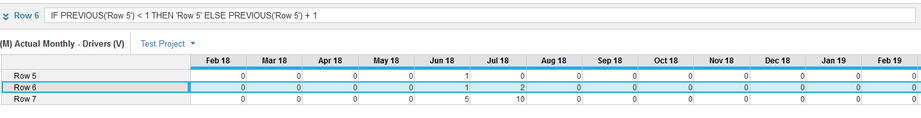Row 6 line item is the one that needs the formula for the SUM in excel.

Appreciate the help

Regards,

Tagged:

•If the values need to be incremental then your formula should be

ELSE (Previous(Row 6) +1)  & not ROW 5

Thanks,

Misbah

•Hi @Jsdeloria21 ,

As @Misbah told to you that In the ELSE Condition you need to put ROW 6 Instead of ROW 5. Please find the below screenshot.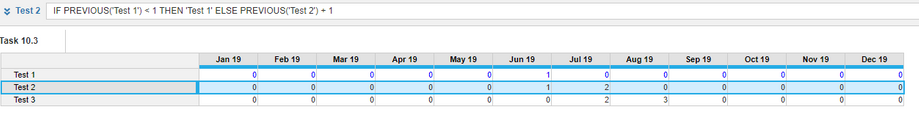Thanks

•Hi Misbah,

Appreciate the help on the issue. I just want to know how can I continue the numbers 1, 2, 3, and so on. Apparently the clients requirement is continuous counting until the end of the time range.

Regards,

•There are a whole host of Anaplan formulas that work with time. As you are trying to sum over a range of months in your excel file I would recommend using the MOVINGSUM formula.

Here is a link to the Anaplan Time and Date Functions Anapedia page where you can get more information:

•To judge from the comments and the pic attached in your second post, I would say that you should use CUMULATE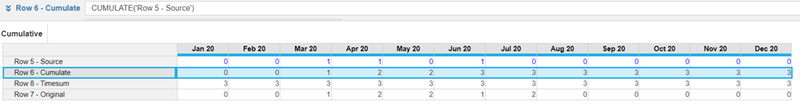CUMULATE will accumulate the "1" along the time period. As you can see, TIMESUM will retrieve the total of the whole time-dimension (unless you specify the beginning and ending of the time range you want to use).

I hope this helps.

Alex.

Olivehorse

•For performance reasons, previous() is faster than Cummulate() in a module using time as previous looks at just the previous member, but cumulate() hits all members over and over.

Rob

•Hi again @Jsdeloria21 ,

In order to meet your need and improve performance as per @rob_marshall  suggestion, you can use the formula shown below: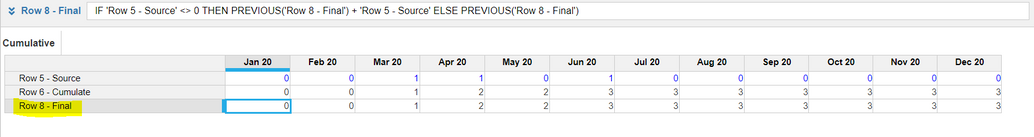As you can see, the output is exactly the same as CUMULATE (which is what I understood you need), but it only uses PREVIOUS.

Let us know if it works

Alex.

•The problem here is with the else part. i.e formula says if previous('Row 5')<1 then 'Row 5' else previous('Row 5')+1.

Else should be greater than 1 according to the formula and hence it would update only July 18 as 2. ( Rest of the time series won't fall under else part)

You can copy the Row 5 value to some dummy line item with the yearvalue() or some formula and apply the logic.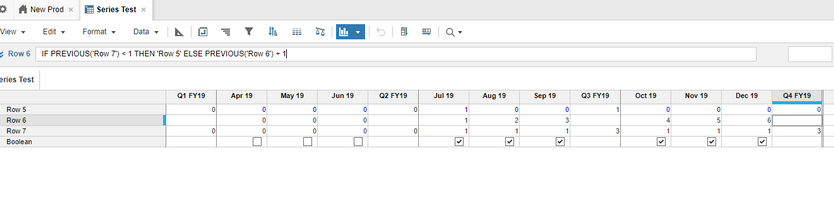Again I am not sure whether it helps in your case.

Thanks,

Sandeep